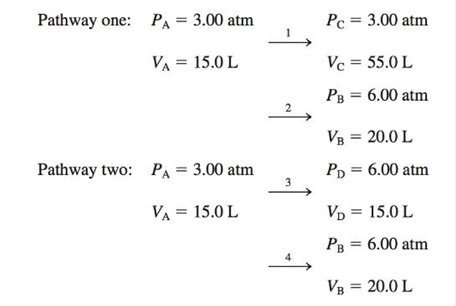# Consider a sample containing 5.00 moles of a monatomic ideal gas that is taken from state A to state B by the following two pathways: For each step, assume that the external pressure is constant and equals the final pressure of the gas for that step. Calculate q, w, ∆E and ∆H for each step in kJ, and calculate overall values for each pathway. Explain how the overall values for the two pathways illustrate that ∆E and ∆H are state functions, whereas q and w are path functions. Hint: In a more rigorous study of thermochemistry, it can be shown that for an ideal gas: Δ E = nC v Δ T and Δ H = nC p Δ T where C v is the molar heat capacity at constant volume and C p is the molar heat capacity at constant pressure. In addition, for a monatomic ideal gas, C v = 3 2 R and C p = 5 2 R , where R = 8.3145 J/K ·mol.### Chemistry: An Atoms First Approach

2nd Edition
Steven S. Zumdahl + 1 other
Publisher: Cengage Learning
ISBN: 9781305079243

#### Solutions

Chapter
Section### Chemistry: An Atoms First Approach

2nd Edition
Steven S. Zumdahl + 1 other
Publisher: Cengage Learning
ISBN: 9781305079243
Chapter 16, Problem 121MP
Textbook Problem
110 views

## Consider a sample containing 5.00 moles of a monatomic ideal gas that is taken from state A to state B by the following two pathways:For each step, assume that the external pressure is constant and equals the final pressure of the gas for that step. Calculate q, w, ∆E and ∆H for each step in kJ, and calculate overall values for each pathway. Explain how the overall values for the two pathways illustrate that ∆E and ∆H are state functions, whereas q and w are path functions. Hint: In a more rigorous study of thermochemistry, it can be shown that for an ideal gas: Δ E = nC v Δ T  and Δ H = nC p Δ T where Cv is the molar heat capacity at constant volume and Cp is the molar heat capacity at constant pressure. In addition, for a monatomic ideal gas, C v = 3 2 R   and     C p = 5 2 R , where R = 8.3145 J/K ·mol.

Interpretation Introduction

Interpretation: The values of q,w,ΔE and ΔH for each of the given steps in the two pathways in the given state change is to be calculated.

Concept Introduction: The internal energy change for the monoatomic ideal gas is calculated by using the formula,

ΔE=32nR(T2T1)

The enthalpy change of the monoatomic ideal gas is calculated by using the formula,

ΔH=52nR(T2T1)

To determine: The values of q,w,ΔE and ΔH for each of the given steps.

### Explanation of Solution

Given

The pathway one for the change in state from A to B is,

PA=3.00atmVA=15.0L1PC=3.00atmVC=55.0LPC=3.00atmVC=55.0L2PB=6.00atmVB=20.0L

The pathway two for the change in state from A to B is,

PA=3.00atmVA=15.0L3PD=6.00atmVD=15.0LPD=6.00atmVD=15.0L4PB=6.00atmVB=20.0L

The number of moles of monoatomic gas in the sample is 5.00mol.

The temperature corresponding to each of the given states is calculated by using the ideal gas equation.

PV=nRTT=PVnR (1)

Where,

• n is the number of moles of the gas.
• P is the absolute pressure of the gas.
• V is the volume of the gas.
• T is the absolute temperature of the gas.
• R is the gas constant.

Substitute the given values of P,V,n and R for state A in the equation (1).

TA=3.00atm×15.00L5.00mol×0.08206Latm/molK=109.68K

Substitute the given values of P,V,n and R for state B in the equation (1).

TB=6.00atm×20.00L5.00mol×0.08206Latm/molK=292.47K

Substitute the given values of P,V,n and R for state C in the equation (1).

TC=3.00atm×55.00L5.00mol×0.08206Latm/molK=402.14K

Substitute the given values of P,V,n and R for state D in the equation (1).

TD=6.00atm×15.00L5.00mol×0.08206Latm/molK=219.35K

The internal energy change for each of the given steps for the monoatomic gas is calculated by using the formula,

ΔE=32nR(T2T1) (2)

Where,

• n is the number of moles of the gas.
• R is the gas constant.
• T2 is the final temperature.
• T1 is the initial temperature.
• ΔE is the internal energy change.

Substitute the values of n , R , T2 and T1 for the step 1 in the equation (2).

ΔE=32×5.00mol×8.3145J/molK×(402.14109.68)K=18237.4J(=18.237kJ)

Substitute the values of n , R , T2 and T1 for the step 2 in the equation (2).

ΔE=32×5.00mol×8

### Still sussing out bartleby?

Check out a sample textbook solution.

See a sample solution

#### The Solution to Your Study Problems

Bartleby provides explanations to thousands of textbook problems written by our experts, many with advanced degrees!

Get Started

Find more solutions based on key concepts
What do the various chromatographic separation techniques have in common?

General Chemistry - Standalone book (MindTap Course List)

Some segments of a protein strand coil, somewhat like a metal spring, because a. amino acids at different place...

Nutrition: Concepts and Controversies - Standalone book (MindTap Course List)

What is meant by biological evolution?

Human Biology (MindTap Course List)

How is seawaters salinity expressed?

Oceanography: An Invitation To Marine Science, Loose-leaf Versin

Suppose you have two eggs, one hard-boiled and the other uncooked. You wish to determine which is the hard-boil...

Physics for Scientists and Engineers, Technology Update (No access codes included)